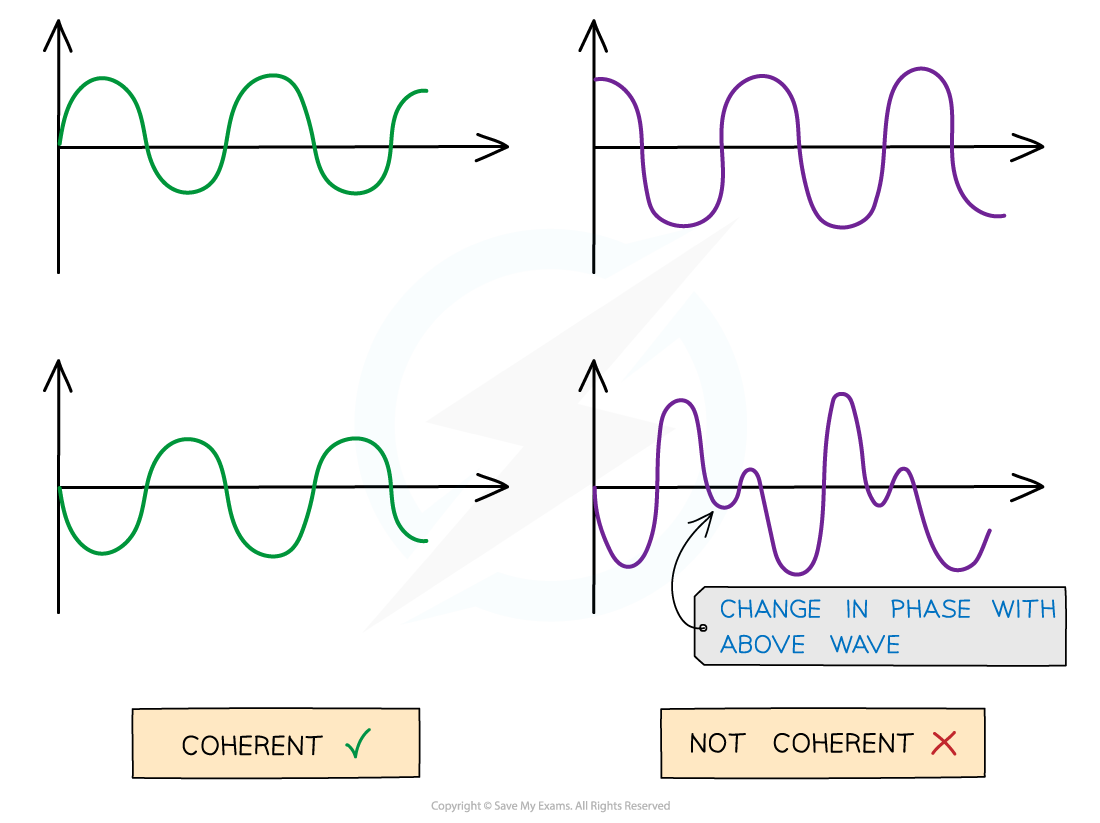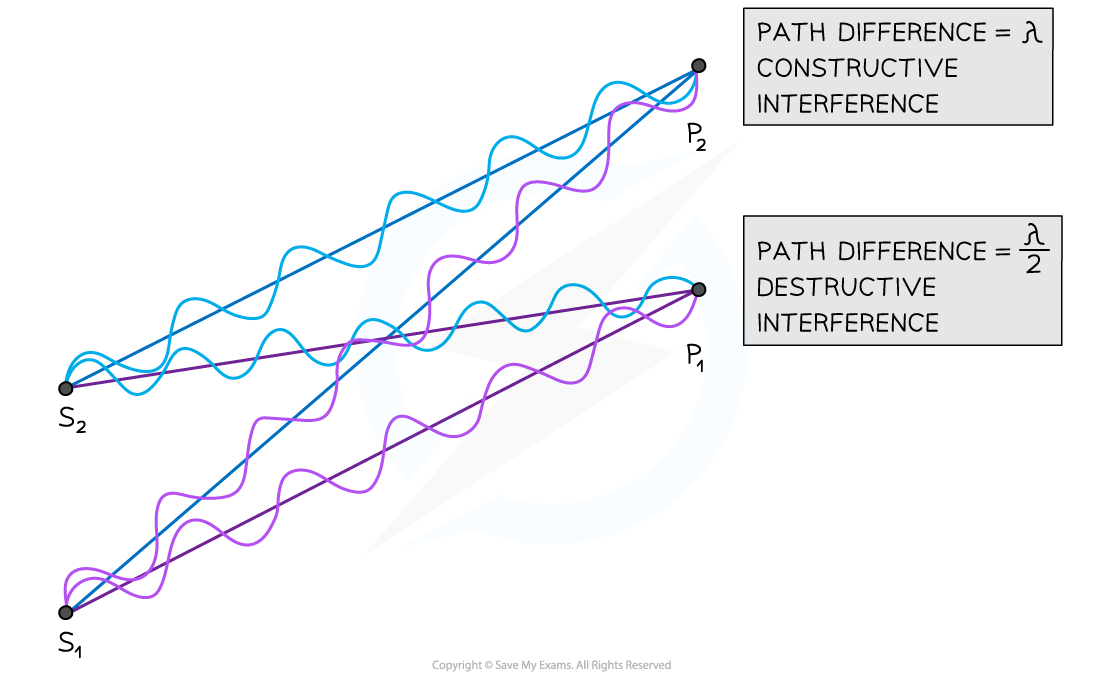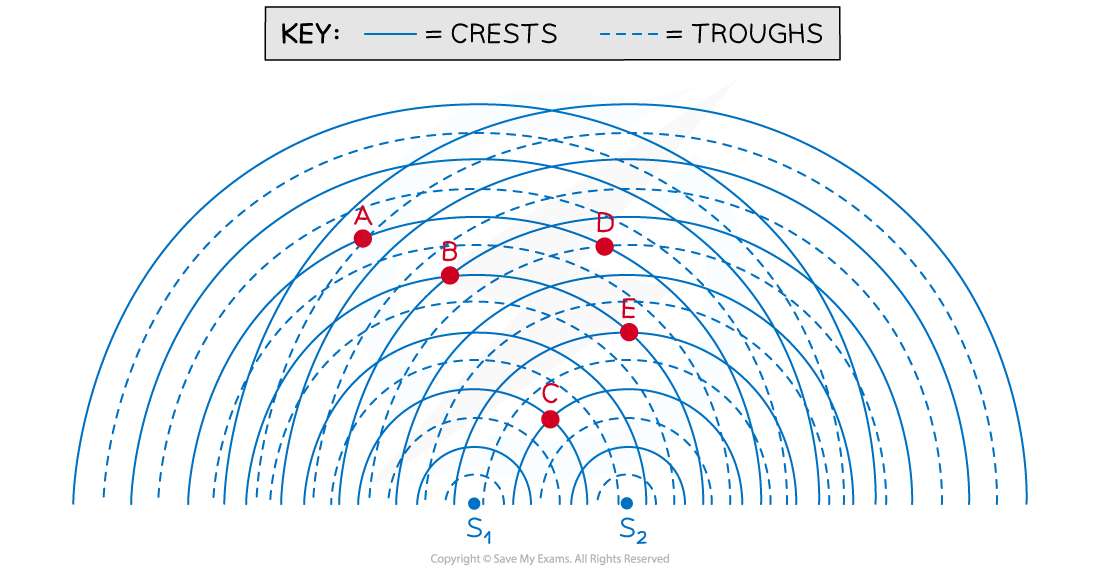# IB DP Physics: SL复习笔记4.4.6 Interference & Path Difference

### Double-Slit Interference

• Interference occurs whenever two or more waves combine to produce a resultant wave with a new resultant displacement
• The waves combine according to the principle of superposition
• Constructive interference happens when the resultant wave has a larger displacement than any of the individual displacements
• Destructive interference happens when the positive displacement of one wave and the negative displacement of another wave exactly cancel out giving a resultant displacement of zero

#### Coherence

• Interference is only observable if produced by a coherent source
• Waves are said to be coherent if they have:
• A constant phase difference
• The same frequencyCoherent waves (on the left) and non-coherent waves (on the right). The abrupt change in phase creates an inconsistent phase difference

• A coherent beam of light contains light waves that are monochromatic and have a constant phase difference
• Monochromatic light consists of light waves of a single frequency
• Laser light is an example of a coherent light source
• Filament lamps produce incoherent light waves

#### Double-Slit Interference of Light

• When a coherent beam of light is incident on two narrow slits very close together, diffraction occurs at each slit (i.e. the waves spread out)
• As the diffracted waves cross, they interfere with each other
• If a screen is placed some distance away from the slits, a pattern of equally spaced bright and dark fringes is observed on the screen
• The bright fringes form where the waves interfere constructively (i.e. a crest meets a crest or a trough meets a trough)
• The dark fringes form where the waves interfere destructively (i.e. a crest meets a trough)Coherent light waves interfere after passing through two narrow slits. Alternating bright and dark fringes are observed on the screen

### Path Difference

• The type of interference occurring at a given point (i.e. constructive or destructive) depends on the path difference of the overlapping waves
• Path difference is defined as:

The difference in distance travelled by two waves from their sources to the point where they meet

• Path difference is generally expressed in multiples of wavelengthAt point P2 the waves have a path difference of a whole number of wavelengths resulting in constructive interference. At point P1 the waves have a path difference of an odd number of half wavelengths resulting in destructive interference

• In the diagram above, the number of wavelengths between:
• S1 ➜ P1 = 6λ
• S2 ➜ P1 = 6.5λ
• S1 ➜ P2 = 7λ
• S2 ➜ P2 = 6λ
• The path difference is:
• (6.5λ – 6λ) = λ / 2 at point P1
• (7λ – 6λ) = λ at point P2
• Hence:
• Destructive interference occurs at point P1
• Constructive interference occurs at point P2

#### Conditions for Constructive and Destructive Interference

• In general, for waves emitted by two coherent sources very close together:
• The condition for constructive interference is:

path difference = nλ

• The condition for destructive interference is:

path difference = (n + ½)λ

• Where:
• λ = wavelength of the waves in metres (m)
• n = 0, 1, 2, 3... (any other integer)
• The same conditions apply to waves emitted by a single coherent source and diffracted by two narrow slits very close together

#### Path Difference and WavefrontsAt point P the waves have a path difference of a whole number of wavelengths resulting in constructive interference

• Another way to represent waves spreading out from two sources is shown in the diagram above
• At point P, the number of crests from:
• Source S1 = 4λ
• Source S2 = 6λ
• The path difference at P is (6λ – 4λ) = 2λ
• This is a whole number of wavelengths (n = 2), hence constructive interference occurs at point P

#### Worked Example

The diagram below is a snapshot of overlapping wavefronts resulting from the interference of coherent waves diffracted by two narrow slits S1 and S2.For each of the points shown, determine:

• The path difference from the sources
• The value of n in the path difference formula
• Whether they are locations of constructive or destructive interference

Step 1: Count the number of wavelengths between each source and the desired point

• E.g. Number of wavelengths between:
• S1 ➜ A = 5λ
• S2 ➜ A = 6.5λ

Step 2: Determine the path difference by subtracting the distances of the point from the two sources

• E.g. Path difference at A = (6.5λ – 5λ) = 1.5λ

Step 3: Compare the path difference calculated in Step 2 with the condition for constructive or destructive interference and give the value of n

• E.g. Path difference at A = 1.5λ = (n + ½)λ ➜ n = 1

Step 4: Decide whether the point is a location of constructive or destructive interference

• E.g. A is a location of destructive interference
• Point A:
• Path difference = (6.5λ – 5λ) = 1.5λ
• n = 1
• Destructive interference
• Point B:
• Path difference = (5λ – 4λ) = λ
• n = 1
• Constructive interference
• Point C:
• Path difference = (2λ – 2λ) = 0
• n = 0
• Constructive interference
• Point D:
• Path difference = (5λ – 4.5λ) = 0.5λ
• n = 0
• Destructive interference
• Point E:
• Path difference = (4λ – 3λ) = λ
• n = 1
• Constructive interference

#### Exam Tip

You are not required to memorise the conditions for constructive and destructive interference, as these are given in the data booklet.You must be able to determine the path difference of waves from two sources (or two narrow slits) at a given point. You can then compare this with the given conditions for constructive and destructive interference, in order to decide which type of interference occurs at the point you are considering.